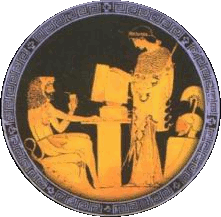##Ελληνική Λίστα Κουίζ (QotD)

### Θέμα: Epanalambanomena rizika (boh8eia)

From: Yannis Chatzigiannelis (cv94044(@)central.ntua.gr)
Date: Παρ 16 Ιουλ 1999 - 14:23:33 EEST

isxyei

(n+k)^2 = n^2 + 2nk +k^2 = 1 + n^2 + 2nk +k^2 -1=

• 1 + n^2 + (k+1+k-1)n + (k+1)(k-1) = 1 + (n+k+1)(n+k-1)

Quiz of the Day ... Ελληνική ίστα με σπαζοκεφαλιές ... και άλλα ...
Πληροφορίες --> https://anekdota.duckdns.org/quiz_list.html

Γραφτείτε και εσείς στην Ελληνική Λίστα με σπαζοκεφαλιές (QotD) και στείλτε τα κουίζ σας!!!

Επιστροφή στον κεντρικό κατάλογο αυτού του αρχείου